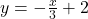## Write the equation of the line 1x + 3y = 6 in y = mx + b form.

Question

Write the equation of the line 1x + 3y = 6 in y = mx + b form.

in progress 0
7 months 2021-07-18T05:32:18+00:00 2 Answers 3 views 0

y = -1/3x + 2

Step-by-step explanation:

1x + 3y = 6

3y = -x + 6

y = -1/3x + 2Step-by-step explanation:

1x + 3y = 6

We want the y to be isolated, so we subtract x from both sides,

3y = 6 – x

Now we divide everything by 3, to isolate y